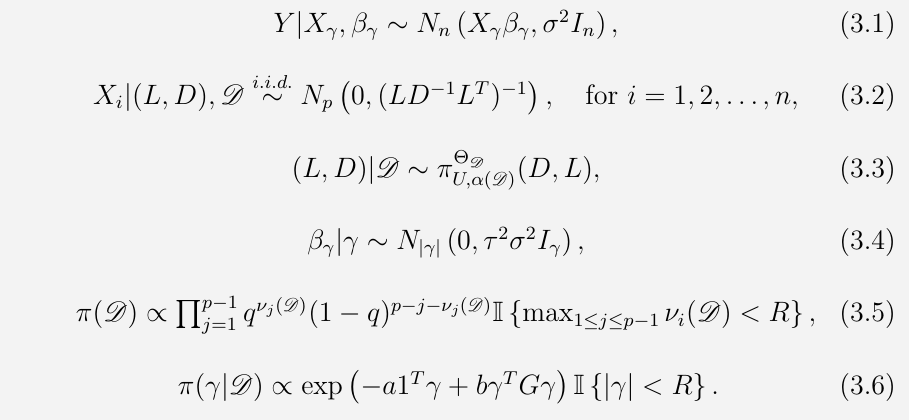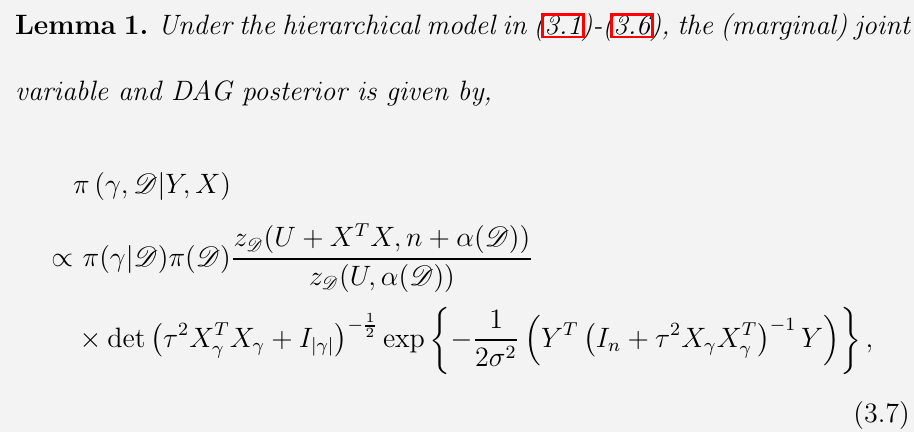# Joint Bayesian Variable and DAG Selection

##### Posted on Oct 31, 2022

joint sparse estimation of regression coefficients and the covariance matrix for covariates in a high-dimensional regression model, where

• the predictors are both relevant to a response variable of interest and functionally related to one another via a Gaussian directed acyclic graph (DAG) model
• Gaussian DAG models introduce sparsity in the Cholesky factor of the inverse covariance matrix, and the sparsity pattern in turn corresponds to specific conditional independence assumptions on the underlying predictors

The paper considers a hierarchical model with spike and slab priors on the regression coefficients and a flexible and general class of DAG-Wishart distributions with multiple shape parameters on the Cholesky factors of the inverse covariance matrix.

under mild regularity assumptions, the paper establishes the joint selection consistency for both the variable and the underlying DAG of the covariates when the dimension of predictors is allowed to grow much larger than the sample size.

## Literature Review

### graph structure is known

• Li and Li (2008, 2010): a graph-constrained regularization procedure and its theoretical properties to take into account the neighborhood information of the variables measured on a known graph.
• Pan et al. (2010): a grouped penalty based on the $L_\gamma$-norm that smooths the regression coefficients of the predictors over the available network
• Li and Zhang (2010), Stingo and Vannucci (2010): incorporate a graph structure in the Markov random field (MRF) prior on indicators of variable selection
• Stingo et al. (2011) and Peng et al. (2013): propose the selection of both pathways and genes within them based on prior knowledge on gene-gene-interactions or functional relationships.

### underlying graph is unknown

• Dobra (2009): estimate a network among relevant predictors by first performing a stochastic search in the regression setting to identify possible subsets of predictors, then applying a Bayesian model averaging method to estimate a dependency network
• Liu et al. (2014): a Bayesian method for regularized regression, which provides inference on the inter-relationship between variables by explicitly modeling through a graph Laplacian matrix.
• Peterson et al. (2016)
• Chekouo et al. (2015) and Chekouo et al. (2016): relate two sets of covariate via a DAG to integrate multiple genomic platforms and select the most relevant features

The goal of the paper: investigate if joint selection consistency results could be established in the high-dimensional regression setting with network-structured predictors

The paper consider a hierarchical multivariate regression model with

• DAG-Wishart priors on the covariance matrix for the predictors
• spike and slab priors on regression coefficients
• independent Bernoulli priors for each edge in the DAG
• a MRF linking the variable indicators to the graph structure

Under high-dimensional settings, establish posterior ratio consistency.

• the strong selection consistency implies that under the true model, the posterior probability of the true variable indicator and the true graph converges in probability to 1 as $n\rightarrow \infty$.

A Gaussian DAG model over a given DAG $\cal D$, denoted by $N_\cD$, consists of all multivariate Gaussian distributions which obey the directed Markov property with respect to a DAG $\cD$. In particular, if $x = (x_1,\ldots,x_p)^T\sim N_p(0,\Sigma)$ and $N_p(0,\Sigma)\in N_\cD$, then $x_i\perp x_{i+1,\ldots,p\backslash pa_i(\cD)}\mid x_{pa_i(\cD)}$ for each $i$.

Any positive definite matrix $\Omega$ can be uniquely decomposed as $\Omega = LD^{-1}L^T$, where $L$ is a lower triangular matrix with unit diagonal entries, and $D$ is a diagonal matrix with positive diagonal entries.

If $\Omega = LD^{-1}L^T$, then $N_p(0, \Omega^{-1})\in N_\cD$ iff $L_{ij}=0$ whenever $i\not\in pa_j(\cD)$.

DAG-Wishart distributions form a conjugate family of priors for the Gaussian DAG model.

• response $Y\in\IR^n \sim N(X\beta,\sigma^2I_n)$
• predictors $X=(X_1,\ldots,X_n)^T\in \IR^{n\times p}$, where $X_i\sim N_p(0, (LD^{-1}L^T)^{-1})$ for $i=1,\ldots,n$Published in categories Note